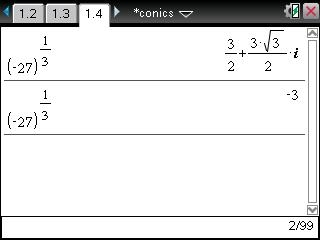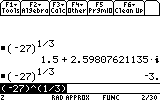# Knowledge Base

## Solution 31051: Unexpected Answer Returned When Calculating Negative Roots Using the TI-Nspire™ Family and TI-89 Family In Rectangular Mode.

### Why do I receive an incorrect answer when I calculate negative roots using my TI-Nspire family handheld or TI-89 family graphing calculator in rectangular mode?

Calculating the cubed root of -27 while in rectangular mode on the TI-Nspire handheld family (operating system version 3.1.0.392) will return the answer of 1.5 + 2.59808 * i. However, calculating the cubed root of -27 while in real mode will return the answer of -3.

The calculator was designed to give these results. When the complex mode is set to rectangular, the calculator is designed to return the principal branch. When the complex mode is set to real, the calculator is designed to return the real root.Calculating the cubed root of -27 while in rectangular mode on the TI-89 family graphing calculator will return the answer of 1.5+2.59808 * i. However, calculating the cubed root of -27 while in real mode will return the answer of -3.Please see the TI-89 family, TI-92 family and Voyage 200 guidebooks for additional information.